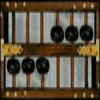Search by Topic

Resources tagged with Place value similar to The Set of Numbers:

Filter by: Content type:
Age range:
Challenge level:

There are 48 results

Broad Topics > Numbers and the Number System > Place valueWhat Number?

Age 5 to 7 Short Challenge Level:

I am less than 25. My ones digit is twice my tens digit. My digits add up to an even number.The Thousands Game

Age 7 to 11 Challenge Level:

Each child in Class 3 took four numbers out of the bag. Who had made the highest even number?Napier's Bones

Age 7 to 11 Challenge Level:

The Scot, John Napier, invented these strips about 400 years ago to help calculate multiplication and division. Can you work out how to use Napier's bones to find the answer to these multiplications?What Do You Need?

Age 7 to 11 Challenge Level:

Four of these clues are needed to find the chosen number on this grid and four are true but do nothing to help in finding the number. Can you sort out the clues and find the number?One of Thirty-six

Age 5 to 7 Challenge Level:

Can you find the chosen number from the grid using the clues?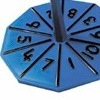Our Numbers

Age 5 to 7 Challenge Level:

These spinners will give you the tens and unit digits of a number. Can you choose sets of numbers to collect so that you spin six numbers belonging to your sets in as few spins as possible?Becky's Number Plumber

Age 7 to 11 Challenge Level:

Becky created a number plumber which multiplies by 5 and subtracts 4. What do you notice about the numbers that it produces? Can you explain your findings?Being Curious - Primary Number

Age 5 to 11 Challenge Level:

Number problems for inquiring primary learners.Being Resourceful - Primary Number

Age 5 to 11 Challenge Level:

Number problems at primary level that require careful consideration.Being Resilient - Primary Number

Age 5 to 11 Challenge Level:

Number problems at primary level that may require resilience.Song Book

Age 7 to 11 Challenge Level:

A school song book contains 700 songs. The numbers of the songs are displayed by combining special small single-digit cards. What is the minimum number of small cards that is needed?Which Is Quicker?

Age 7 to 11 Challenge Level:

Which is quicker, counting up to 30 in ones or counting up to 300 in tens? Why?Which Scripts?

Age 7 to 11 Challenge Level:

There are six numbers written in five different scripts. Can you sort out which is which?Age 5 to 11 Challenge Level:

Try out this number trick. What happens with different starting numbers? What do you notice?Oddly

Age 7 to 11 Challenge Level:

Find the sum of all three-digit numbers each of whose digits is odd.Six Is the Sum

Age 7 to 11 Challenge Level:

What do the digits in the number fifteen add up to? How many other numbers have digits with the same total but no zeros?ABC

Age 7 to 11 Challenge Level:

In the multiplication calculation, some of the digits have been replaced by letters and others by asterisks. Can you reconstruct the original multiplication?Diagonal Sums

Age 7 to 11 Challenge Level:

In this 100 square, look at the green square which contains the numbers 2, 3, 12 and 13. What is the sum of the numbers that are diagonally opposite each other? What do you notice?Multiply Multiples 3

Age 7 to 11 Challenge Level:

Have a go at balancing this equation. Can you find different ways of doing it?Multiply Multiples 1

Age 7 to 11 Challenge Level:

Can you complete this calculation by filling in the missing numbers? In how many different ways can you do it?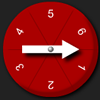Two Spinners

Age 5 to 7 Challenge Level:

What two-digit numbers can you make with these two dice? What can't you make?Light the Lights

Age 5 to 7 Challenge Level:

Investigate which numbers make these lights come on. What is the smallest number you can find that lights up all the lights?Writing Digits

Age 5 to 7 Challenge Level:

Lee was writing all the counting numbers from 1 to 20. She stopped for a rest after writing seventeen digits. What was the last number she wrote?Spell by Numbers

Age 7 to 11 Challenge Level:

Can you substitute numbers for the letters in these sums?Being Collaborative - Primary Number

Age 5 to 11 Challenge Level:

Number problems at primary level to work on with others.Nice or Nasty for Two

Age 7 to 14 Challenge Level:

Some Games That May Be Nice or Nasty for an adult and child. Use your knowledge of place value to beat your opponent.All the Digits

Age 7 to 11 Challenge Level:

This multiplication uses each of the digits 0 - 9 once and once only. Using the information given, can you replace the stars in the calculation with figures?Trebling

Age 7 to 11 Challenge Level:

Can you replace the letters with numbers? Is there only one solution in each case?One Million to Seven

Age 7 to 11 Challenge Level:

Start by putting one million (1 000 000) into the display of your calculator. Can you reduce this to 7 using just the 7 key and add, subtract, multiply, divide and equals as many times as you like?Round the Three Dice

Age 7 to 11 Challenge Level:

What happens when you round these three-digit numbers to the nearest 100?Multiply Multiples 2

Age 7 to 11 Challenge Level:

Can you work out some different ways to balance this equation?Number Detective

Age 5 to 11 Challenge Level:

Follow the clues to find the mystery number.Coded Hundred Square

Age 7 to 11 Challenge Level:

This 100 square jigsaw is written in code. It starts with 1 and ends with 100. Can you build it up?Subtraction Surprise

Age 7 to 14 Challenge Level:

Try out some calculations. Are you surprised by the results?Age 5 to 11 Challenge Level:

Who said that adding couldn't be fun?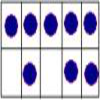Number Sense Series: A Sense of 'ten' and Place Value

Age 5 to 7

Once a basic number sense has developed for numbers up to ten, a strong 'sense of ten' needs to be developed as a foundation for both place value and mental calculations.Age 7 to 14 Challenge Level:

Watch our videos of multiplication methods that you may not have met before. Can you make sense of them?Round the Dice Decimals 2

Age 7 to 11 Challenge Level:

What happens when you round these numbers to the nearest whole number?Round the Dice Decimals 1

Age 7 to 11 Challenge Level:

Use two dice to generate two numbers with one decimal place. What happens when you round these numbers to the nearest whole number?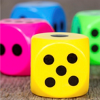Dicey Operations in Line for Two

Age 7 to 11 Challenge Level:

Dicey Operations for an adult and child. Can you get close to 1000 than your partner?The Number Jumbler

Age 7 to 14 Challenge Level:

The Number Jumbler can always work out your chosen symbol. Can you work out how?Alien Counting

Age 7 to 11 Challenge Level:

Investigate the different ways these aliens count in this challenge. You could start by thinking about how each of them would write our number 7.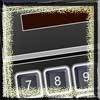Calculator Bingo

Age 7 to 11 Challenge Level:

A game to be played against the computer, or in groups. Pick a 7-digit number. A random digit is generated. What must you subract to remove the digit from your number? the first to zero wins.Reach 100

Age 7 to 14 Challenge Level:

Choose four different digits from 1-9 and put one in each box so that the resulting four two-digit numbers add to a total of 100.Path of Discovery Series 3: I Do and I Understand

Age 5 to 7

Marion Bond recommends that children should be allowed to use 'apparatus', so that they can physically handle the numbers involved in their calculations, for longer, or across a wider ability band,. . . .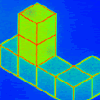Pupils' Recording or Pupils Recording

Age 5 to 14

This article, written for teachers, looks at the different kinds of recordings encountered in Primary Mathematics lessons and the importance of not jumping to conclusions!